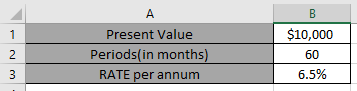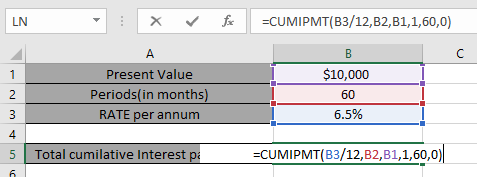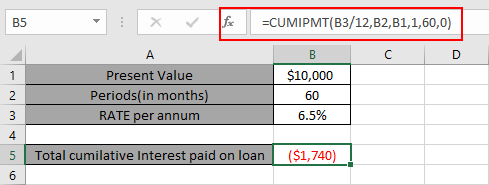# How to use the Excel CUMIPMT function

In this article, we will learn how to use the CUMIPMT function to calculate Cumulative Interest paid from the start_period and end_period in Excel.

In simple words, Excel let’s a person find his cumulative interest paid in installments on a loan amount using the function having present value or loan amount, interest rate per month and the period of payment.

The function calculates the Cumulative Interest for a loan based on constant payments and a constant interest rate.
Syntax:

=CUMIPMT (rate, nper, pv, start_period, end_period, [type])

Syntax for loan calculation formula:-
Rate – The interest rate per period.
Nper – The total number of payments.
Pv – The present value or the loan amount
Start_period : Interest amount paid for the start period.
End_period : Interest amount paid for the end period
Type – The timing of the payment, either at the beginning or end of the period. Numbers 0 or 1 represent the payment date. The number 0 represents payment at the end of the period, and the number 1 represents payment at the beginning of the period. The default (empty argument) is 0. The calculation is at the end of the period.

All of the above might be confusing for some people, so let’s gear up & start learning its usage in excel with the example.

Consider a scenario, John has taken a loan of \$10,000 from a bank at 6.5% per year interest in 5 years period. He needs to find out his cumulative Interest paid for the period on the loan.

So he added the following details in Excel.Use the formula in B6 cell

=CUMIPMT(B3/12, B2, B1, 1, 60, 0)

B3/12 : rate per annum
B2 : total payment period
B1 : the loan amount (taken in positive)
1 : start_period
60 : end_period (for cumulative Interest the whole period will be included)You must be wondering why Interest rate is divided by 12 as Applying the formula in the cell.John has to pay \$1,740 over the loan as Interest. You must be wondering why this amount shown here is negative. The loan amount is positive which is credited to John. He has to pay that amount which is to be debited.

Hope you understood how to use the CUMIPMT function to calculate Cumulative Interest paid from the start_period and end_period in Excel. Explore more articles here on calculating account functions here. Please state your queries in the comment box below.

Related Articles:

How to use the DURATION Function in Excel

How to use the Excel IPMT Function

How to Use Compound Interest Function in Excel

Popular Articles:

How to use the VLOOKUP Function in Excel

How to use the COUNTIF function in Excel 2016

How to Use SUMIF Function in Excel

Terms and Conditions of use

The applications/code on this site are distributed as is and without warranties or liability. In no event shall the owner of the copyrights, or the authors of the applications/code be liable for any loss of profit, any problems or any damage resulting from the use or evaluation of the applications/code.